# How to Split Text String to an Array

This post explains that how to convert the text to an Array in excel. How to convert text into array using formula in excel 2013? How to split the text string into an array where the each character in text is an element in array using excel formula in excel 2013. How to split text into an array using user defined function in VBA.

## How to split text string into an Array with excel formula

If you want to convert a text string into an array that split each character in text as an element, you can use an excel formula to achieve this result. the below will guide you how to use a combination of the MID function, the ROW function, the INDIRECT function and the LEN function to split a string in Cell B1 into an array in Cell C1. Refer to the below formula:

`{=MID (B1, ROW ( INDIRECT ("1:" &LEN (B1))),1 )}`

Note: the above formula is an array formula, so when you enter it in Cell Ce, you should enter the shortcut “CTRL”+”SHIFT” +”enter” to indicate that it is an array formula.

Let’s see how the above formula works:

Assuming that the length of the Cell B1 is 5.

=LEN(B1)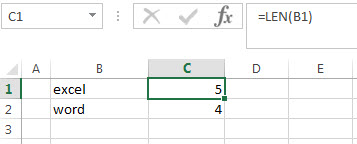The LEN function returns the length of a text string in Cell B1. In other words, you will get the numbers of characters in Cell B1.

=”1:”&LEN(B1)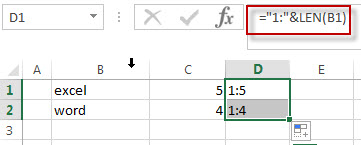The above formula will join the text string between “1:” and the result returned by the LEN function using ampersand operator. it will return a text string as : “1:5”

=INDIRECT(“1:”&LEN(B1))

The INDIRECT returns a cell reference from a specified text string. So it will return the reference from row 1 to row5.

=ROW(INDIRECT(“1:”&LEN(B1)))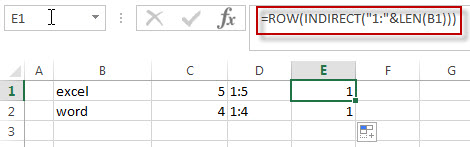You can press “F9” to check the actual element of array returned by the ROW function.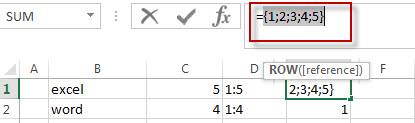The ROW function will return the row number based on the row references returned by the above INDIRECT function. so it will return the below array( each row number is an element in array).

`{1;2;3;4;5}`

=MID(B1, ROW(INDIRECT(“1:”&LEN(B1))),1 )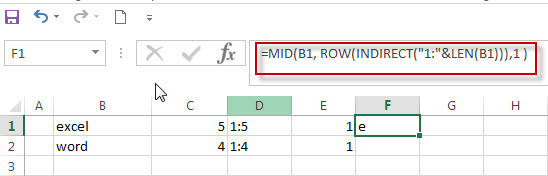You can select the above formula, then press “F9” to get the actual array result.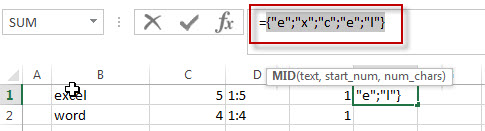The MID function will extract sub strings based on each start_num in array element returned by the above ROW function and the number of character to extract is always 1. So the above MID function will return the below string array in array formula.

`{"e";"x";"c";"e;";"l"}`

## How to split text string into an Array with VBA code

You can also convert the text string to array using a user defined function in VBA. You can follow the below steps to create a new Excel function to split text string into an array in Excel VBA:

1# click on “Visual Basic” command under DEVELOPER Tab.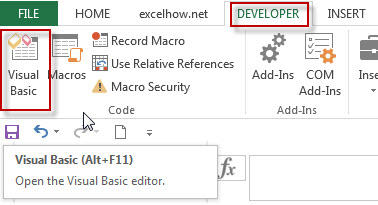2# then the “Visual Basic Editor” window will appear.

3# click “Insert” ->”Module” to create a new module named as: CharToArray1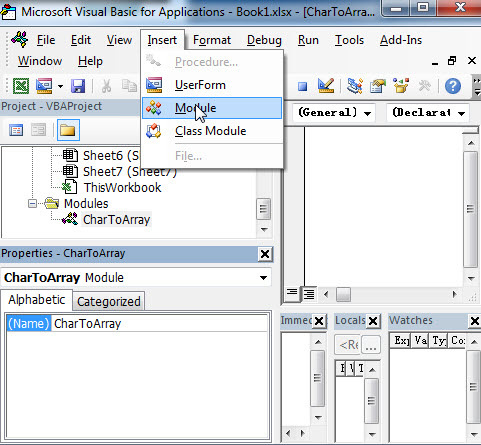4# paste the below VBA code into the code window. Then clicking “Save” button.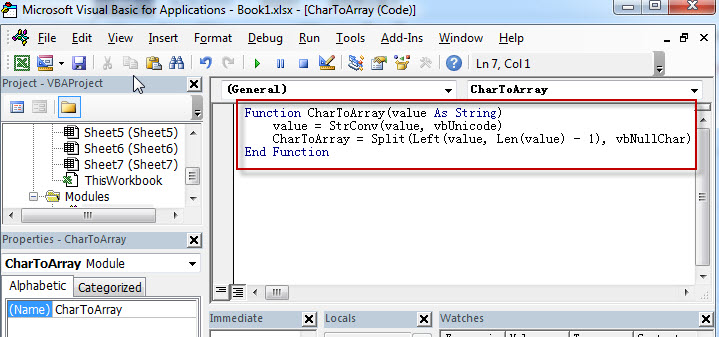```Function CharToArray(value As String)
value = StrConv(value, vbUnicode)
CharacterArray = Split(Left(value, Len(value) - 1), vbNullChar)
End Function```

5# back to the current workbook, then enter the below formula in Cell C1:

`=CharToArray(B1)`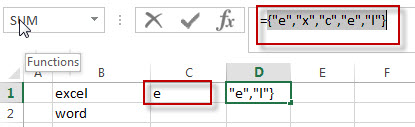### Related Formulas

• Combine Text from Two or More Cells into One Cell
If you want to combine text from multiple cells into one cell and you can use the Ampersand (&) symbol.If you are using the excel 2016, then you can use a new function TEXTJOIN function to combine text from multiple cells…
• Find the Relative Position in a Range or Table
If you want to know the relative row position for all rows in an Excel Range (B3:D6), you can use a excel Array formula as follows:=ROW(B3:D6)- ROW(B3) + 1. You can also use another excel array formula to get the same result as follows:=ROW(B3:D6)-ROW(INDEX(B3:D6,1,1))+1…

### Related Functions

• Excel MID function
The Excel MID function returns a substring from a text string at the position that you specify.The syntax of the MID function is as below:= MID (text, start_num, num_chars)…
• Excel ROW function
The Excel ROW function returns the row number of a cell reference.The ROW function is a build-in function in Microsoft Excel and it is categorized as a Lookup and Reference Function.The syntax of the ROW function is as below:= ROW ([reference])….
• Excel INDIRECT  function
The Excel ROW function returns the row number of a cell reference.The ROW function is a build-in function in Microsoft Excel and it is categorized as a Lookup and Reference Function.The syntax of the ROW function is as below:= ROW ([reference])….
• Excel LEN function
The Excel LEN function returns the length of a text string (the number of characters in a text string).The LEN function is a build-in function in Microsoft Excel and it is categorized as a Text Function.The syntax of the LEN function is as below:= LEN(text)…